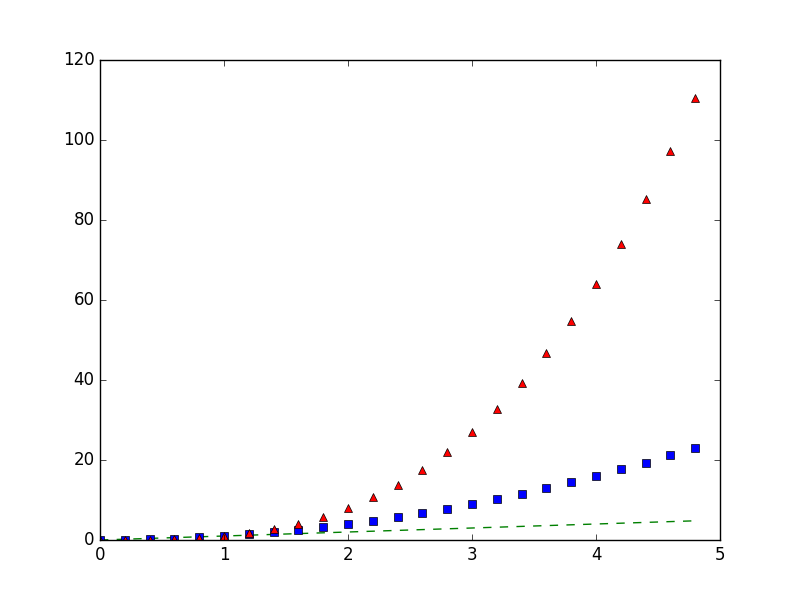﻿ Matplotlib Basic: Plot several lines with different format styles in one command using arrays - w3resource# Matplotlib Basic: Plot several lines with different format styles in one command using arrays

## Matplotlib Basic: Exercise-11 with Solution

Write a Python program to plot several lines with different format styles in one command using arrays.

Sample Solution:

Python Code:

``````import numpy as np
import matplotlib.pyplot as plt

# Sampled time at 200ms intervals
t = np.arange(0., 5., 0.2)

# green dashes, blue squares and red triangles
plt.plot(t, t, 'g--', t, t**2, 'bs', t, t**3, 'r^')
plt.show()
```
```

Sample Output:Python Code Editor: